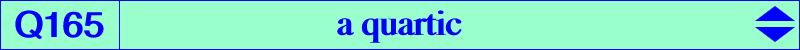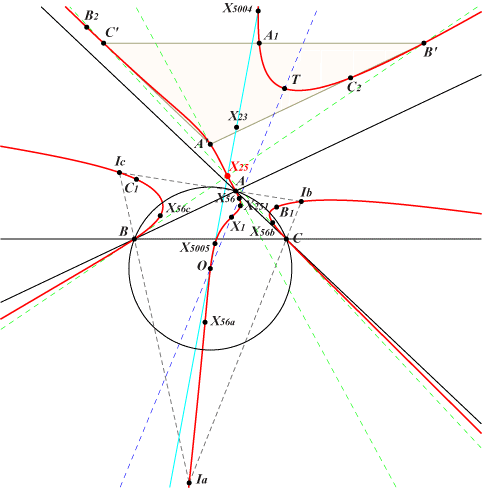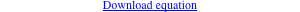too complicated to be written here. Click on the link to download a text file.X(1), X(3), X(25), X(56), X(251), X(5004), X(5005) T = X(40910) excenters X56a, X56b, X56c : extraversions of X(56) extraversions of X(40910) other points belowLet P be a point with circumcevian triangle PaPbPc. Denote by Ea, Eb, Ec the centers X(25) of triangles PaBC, PbCA, PcAB respectively. Recall that X(25) is the barycentric product X(4) x X(6). PaPbPc and EaEbEc are perspective if and only if P lies on the quartic Q165 (together with the circumcircle). Other points on Q164 : • A', B', C' : images of A, B, C under the homothety h(X25, k) where k = 1 / (2 cosA cosB cosC). • A1, B1, C1 and A2, B2, C2 : intersections of the sidelines of A'B'C' with the internal and external bisectors of ABC respectively. • T = a^2 [b^2 sb + c^2 sc - (a^2 + b c) sa] : : on the lines {X1,X3}, {X9,X1486}, {X25,X200}, {X42,X251}, {X100,X2725}, {X101,X2340}, {X105,X3008}, SEARCH = -6.44219214130630. T is now X(40910) in ETC (2021-01-03). • Ta, Tb, Tc : extraversions of T. Ta lies on the line passing through X(3), Ia, X56a and Tb, Tc likewise. See the related quartics Q162, Q163 and Q164. See Q023 for a generalization.